# A brief introduction to diffusion weighted MRI (DWMRI) processing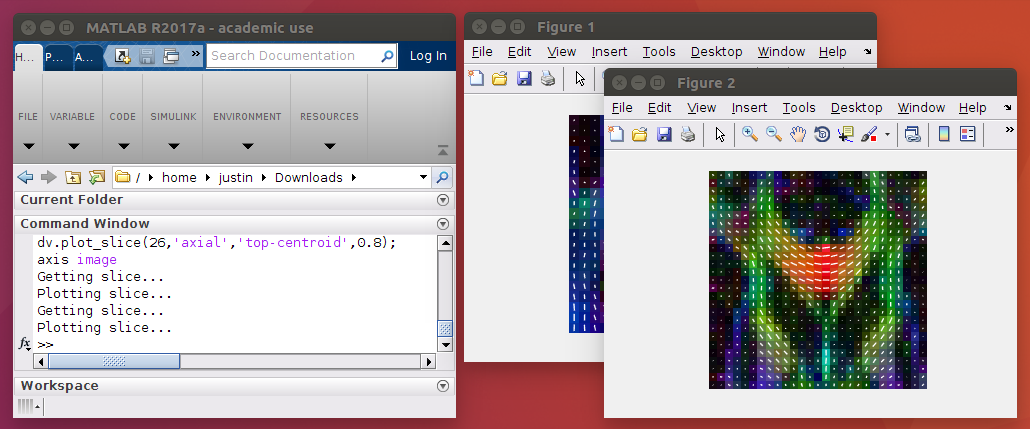This post serves as a brief introduction to DWMRI processing.

dwmri.dcm is a 32 direction, 1000 b-value DWMRI dicom file, dcm2niix is a commonly used DICOM to NIFTI converter, nifti_utils is a library to work with NIFTIs in MATLAB, dwmri_visualizer is a visualization tool for diffusion images in MATLAB, and FSL is a powerful and commonly used medical image processing library.

A very common pipeline for dwmri processing is: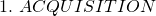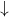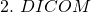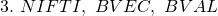1. The first consideration is to determine which acquisition parameters to use; this is application dependent. For example, if diffusion tensor imaging (DTI) is desired, then an acquisition with 32 gradient directions and a b-value of 1000 can be used. For high angular resolution diffusion imaging (HARDI), 60 gradient directions and a b-value of 2000 or greater can be used. Regardless of the desired application, I would recommend acquiring data which makes use of FSL‘s preprocessing tools: topup and eddy. The bare minimum requirement to use both of these tools is to acquire an additional reverse phase-encoded B0 along with your acquisition.
2. and 3. Typically, the output from a diffusion scan is a DICOM file. This format is generally used within a PACs to send data from a scanner to some storage archive. DICOMs typically aren’t used directly in image processing. Instead, NIFTIs are a commonly used. To convert the DICOM in this tutorial to a NIFTI you can use the dcm2niix tool:
```mkdir dwmri_nifti
dcm2niix -f dwmri -o dwmri_nifti/ dwmri.dcm

```

Be sure to use the most updated version of `dcm2niix`. It is actively maintained and new patches are released frequently. The outputs are: `dwmri_ADC.nii, dwmri.bval, dwmri.bvec, and dwmri.nii`. The DICOM in this tutorial came from a Philips scanner which synthesizes an ADC image which is not useful, so we can go ahead and remove `dwmri_ADC.nii`. The `bvec` and `bval` files are metadata containing the gradient directions and diffusion weighting. You can perform some preliminary checks to make sure outputs look correct:

```cat dwmri_nifti/dwmri.bval
cat dwmri_nifti/dwmri.bval | tr '\t' '\n' | wc -l
fslhd dwmri_nifti/dwmri.nii | grep dim[0-9] | grep -v pix

```

You can also view the data in an image viewer like MIPAV or directly in MATLAB.One important aspect of `dcm2niix` is that it outputs the NIFTI and b-vectors in a way which are compatible with FSL. The way it does this is by doing two things: 1) The output NIFTI should have “RADIOLOGICAL” orientation and 2) the b-vectors should be in “RADIOLOGICAL voxel convention“. A NIFTI is in “RADIOLOGICAL” convention if the determinant of its `sform`/`qform` is negative (this essentially means it uses a left-handed coordinate system). b-vectors are in “RADIOLOGICAL voxel convention” if, after the NIFTI has been loaded into “RADIOLOGICAL” convention, the b-vectors are oriented with respect to the voxels. This is super confusing, but suffice it say two things for now: 1) if you use `dcm2niix` your NIFTI and b-vectors will be compatible with FSL and 2) your b-vectors will be oriented with respect to the voxels. Anyway, you can check the NIFTI by using:

```    fslorient dwmri_nifti/dwmri.nii

```

The output should be ` RADIOLOGICAL`. Checking if the b-vectors are in “RADIOLOGICAL voxel convention” is done easiest by fitting a diffusion tensor and plotting the primary eigenvector direction (done in step 5).

3. Preprocessing is pretty involved. There are three main sources of distortions in DWMRI: 1) susceptibility distortions, 2) eddy current distortions and 3) patient movement. The current state of the art is to use FSL’s `topup` and `eddy` which corrects for all three of these things. Prior to this, corrections for eddy currents and patient movement were done using 12-DOF affine registrations (FSL’s ` eddy_correct`, which is now deprecated) while susceptibility distortion was corrected by using a field map. Because `topup`/`eddy` are somewhat complicated, I’ve written an entire article for it. If you are following along and eager to start processing, then just use the raw data for now.
4. Processing! This is the fun part! There are two approaches I’d like to take here: 1) A simple command-line DTI fit and 2) A MATLAB script to perform a DTI fit. DTI is a good way to get introduced to DWMRI processing because it’s a simple model that requires solving linear equations. The b-vector based diffusion equation for DTI is: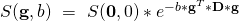Where S is the intensity value at a voxel for a given b-vector and b-value, g is the b-vector, b is the b-value, and D is the diffusion tensor. We have S(g,b), S(0,0), b, and g. We just need to obtain D. If you divide by S(0,0), take the natural log, divide by -b, then solve for the components of D, you will obtain a linear set of equations which you can get the least squares solution to.

Anyway, to get the diffusion tensor fit with FSL, do the following:

```fslroi dwmri_nifti/dwmri.nii dwmri_nifti/b0.nii 0 1
mkdir fsl_dtifit
fslview fsl_dtifit/dti_FA.nii.gz fsl_dtifit/dti_V1.nii.gz

```

The outputs should be like the following (after changing DTI display options to “Lines RGB”):Checking the fibre orientations along the corpus collosum is a standard check to make sure everything is “ok” (or at least not egregiously wrong).

Ok, so doing this from the command-line is cool, but doing it in MATLAB is more powerful. The following will fit the diffusion tensor, compute the main eigenvector (V1) and the fractional anisotropy (FA):

```%% Set environment

%% Fit diffusion tensor

% Split into B0 and DWI
b0_vol = dwmri_vol(:,:,:,bvals == 0);
dwi_vol = dwmri_vol(:,:,:,bvals ~= 0);
bvecs_dwi = bvecs(:,bvals ~= 0);
bvals_dwi = bvals(bvals ~= 0);

% Make output directory
mkdir('matlab_dtifit');

% Loop over and compute diffusion tensor, V1, and FA
V1_vol = zeros(size(dwmri_vol,1),size(dwmri_vol,2),size(dwmri_vol,3),3);
FA_vol = zeros(size(dwmri_vol,1),size(dwmri_vol,2),size(dwmri_vol,3));
for i = 1:size(dwmri_vol,1)
for j = 1:size(dwmri_vol,2)
for k = 1:size(dwmri_vol,3)
% Compute A: -b*g*g'
gx = bvecs_dwi(1,:)';
gy = bvecs_dwi(2,:)';
gz = bvecs_dwi(3,:)';
A = -bsxfun(@times,bvals_dwi',[gx.^2 2*gx.*gy 2*gx.*gz gy.^2 2*gy.*gz gz.^2]);

% Fit using linear least squares
DT = mldivide(A,squeeze(log(abs(dwi_vol(i,j,k,:)./b0_vol(i,j,k)))));
DT_mat = zeros(3,3);

% Reshape to matrix
DT_mat(1,1:3) = DT(1:3);
DT_mat(2,2:3) = DT(4:5);
DT_mat(3,3) = DT(6);
DT_mat(2,1) = DT_mat(1,2);
DT_mat(3,1:2) = DT_mat(1:2,3)';

if all(isfinite(DT_mat(:)))
% Get eigenvectors and eigenvalues
[V,D] = eig(DT_mat);

% Sort by size
[D,I] = sort(diag(D),'descend');
V = V(:, I);

% Store V1
V1_vol(i,j,k,:) = V(:,1);

% Compute FA
FA_vol(i,j,k) = sqrt(1/2)*sqrt((D(1)-D(2))^2 + (D(2)-D(3))^2 + (D(3)-D(1))^2)/sqrt(D(1)^2+D(2)^2+D(3)^2);
end
end
end
end
end

%% Save output

% V1
template_nii.img = V1_vol;
nifti_utils.save_untouch_nii_using_scaled_img_info(fullfile('matlab_dtifit','dti_V1.nii.gz'),template_nii,'double');

% FA
template_nii.img = FA_vol;
nifti_utils.save_untouch_nii_using_scaled_img_info(fullfile('matlab_dtifit','dti_FA.nii.gz'),template_nii,'double');

%% Plot

% Get visualizer
xform_RAS = nifti_utils.get_voxel_RAS_xform(fullfile('dwmri_nifti','dwmri.nii'));
dv = dwmri_visualizer({V1_vol,FA_vol}, ...
[], ...
FA_vol, ...
xform_RAS, ...
'colorized_FA', ...
{'w',1});

% Plot
dv.plot_slice(47,'coronal','top-centroid',1);
axis image
dv.plot_slice(26,'axial','top-centroid',0.8);
axis image

```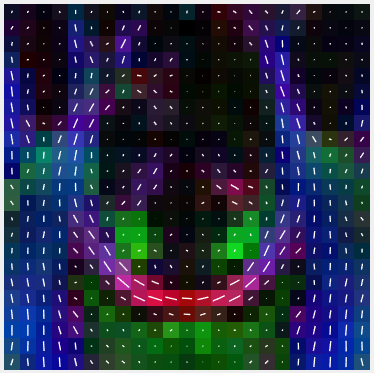The outputs look very similar to the FSL outputs (a good thing):

A few things:

Yes

### Why was I able to load the NIFTI untouched and the b-vectors directly and compute the V1 in the correct orientation?

I was able to do this because `dcm2niix` will output b-vectors with respect to the voxels, which is what my code assumes.

### Is the way FSL deals with b-vectors confusing to anyone else?

Yes. In fact, this resulted in a software bug in the MRtrix package. It most likely went unnoticed for a while because most people use `dcm2niix` and do not have DWMRI NIFTIs in “NEUROLOGICAL” convention, so it was probably assumed that FSL b-vectors were always with respect to voxels, which is only true if the NIFTI is in “RADIOLOGICAL” convention.

### Why does FSL do this!?

I believe it’s because FSL wants to ensure a left-handed coordinate system for all NIFTIs loaded into FSL. This would ensure something like a 6-DOF registration can always be achieved with a rotation and translation. If anyone else knows a better or more correct reason then they can leave a response in the comments.